Courses
Courses for Kids
Free study material
Free LIVE classes
More

# 3D - Geometry(Solid Geometry)## Introduction to Solid Geometry

In geometry, the figure that deals with three-dimensional shapes is known as a 3D shape or a solid shape.

The conventional name for the geometry of three-dimensional Euclidean spaces (also known as 3D geometry) in mathematics is "solid geometry," sometimes known as "stereometry."

Measurements of the volumes of different solid forms (or 3D shapes), such as cylinders, pyramids, prisms, and other polyhedrons, cones, truncated cones, and balls enclosed by spheres, are the subject of stereometry. The whole concept is known as solid geometry. In solid geometry, there are so many types of solids.

## Solid Geometry Definition:

Solids come in many different varieties in terms of geometry. Because they have three dimensions, such as length, breadth, and height, solids are three-dimensional forms. Solids are the bodies that occupy space.

## Terms of Solid Geometry:

• Point: Point has no dimension, it has position only.

• Line: A line has only length. It refers to one dimension.

• Surface: A surface contains length and breadth, and it has two dimensions.

• Solid: A solid has all such as length, breadth, and thickness or height.

For example, the book is known as solid geometric because it contains 6 faces such as a surface; each of its edges is called a line and each corner is considered a point.Book

The following are a few illustrations of solid or three-dimensional shapes:

• Cubes: A cube is a 3-D object. six-sided solid form with six faces. One of the most basic forms in three dimensions is the cube. A cube's six faces are all squares, making it a two-dimensional object.

• Cuboids: A solid with six rectangular faces that are perpendicular to one another.

• Rectangular Solids: A quadrilateral with parallel sides that are equal to one another and four equal vertices is known as a rectangle.

• Prism: A prism is a solid object with flat sides or surfaces, a uniform cross-section throughout its length, and two identical ends (such as a triangle, square, rectangle, etc.).

• Cylinders: A cylinder is a three-dimensional solid object with two bases that are identically circular and are connected by a curving surface that is located at a certain height from the centre.

• Spheres: A sphere is a three-dimensional object that is round in shape.

• Cones: A cone is a three-dimensional geometric structure with a smooth transition from a flat, generally circular base to the vertex, a point that creates an axis to the base's centre.

• Pyramids: A three-dimensional form is a pyramid. A pyramid's flat triangular sides unite at a common point known as the apex and have a polygonal base.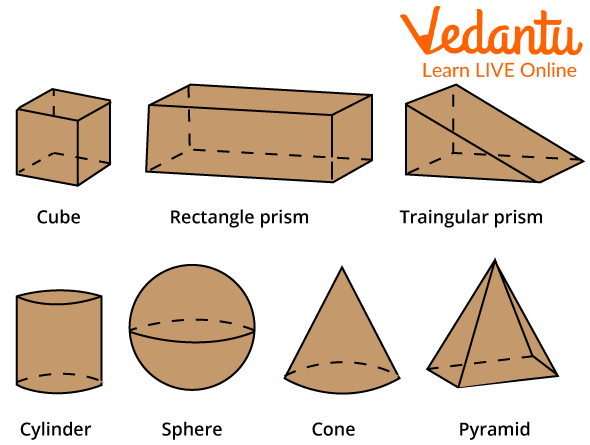Examples of Geometric Shapes

## A Geometric Solid has Only Length and Height:

In solid geometry, shapes contain length, breadth, and height. But there are few shapes that we can consider geometric solids with only length and height. Here, the cone, sphere, and cylinder are also known as solid shapes in geometry. These shapes contain only length and height. In cone or cylinder shapes, there are only lengths, radii, and heights.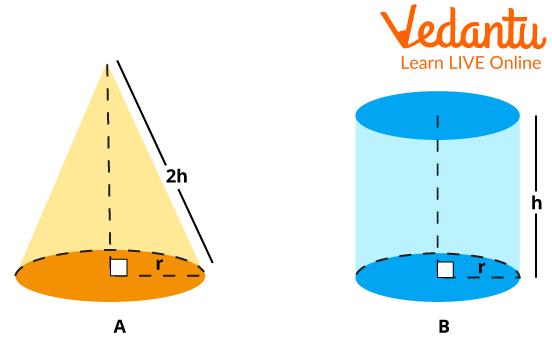Length and Height of a Figure

In a sphere shape, there will be only one radius, which is used as length or height. The reason for this is that because the sphere is round, it does not have the same height and length as other shapes. So to calculate the area, perimeter, etc., of the sphere, only the radius measurement is used. As we can see in the image below, we can only use the radius and diameter as measurements.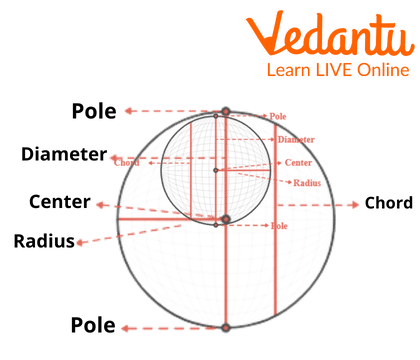Sphere

## Solid Geometry Graph Line:

A graph line is a type of chart used to show some information. Here we study geometrical shapes in graphs. When we represent solid space in.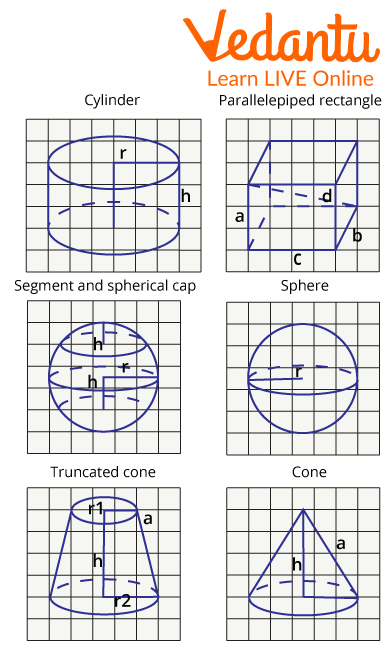Solid Geometry Graph Line

In graphs, we can usually represent the 3 axes in each graph as x, y, and z in three-dimensional space for solid geometry. The geometry is shown in the graph to demonstrate all three angles, as we have already discussed that the solid is three-dimensional, and to make it clearer and easier to understand. Solid geometry is useful in graphs.

## Solved Examples:

1. Define the total faces and lines in a pyramid.

Ans: Faces in Pyramids = 5

Lines in a pyramid = 8

2. What are the faces, lines, and points in a Cuboid?

Ans: Faces in Cuboid = 6

Lines in Cuboid = 12

Points in Cuboid = 8

3. Match the following:

Ans: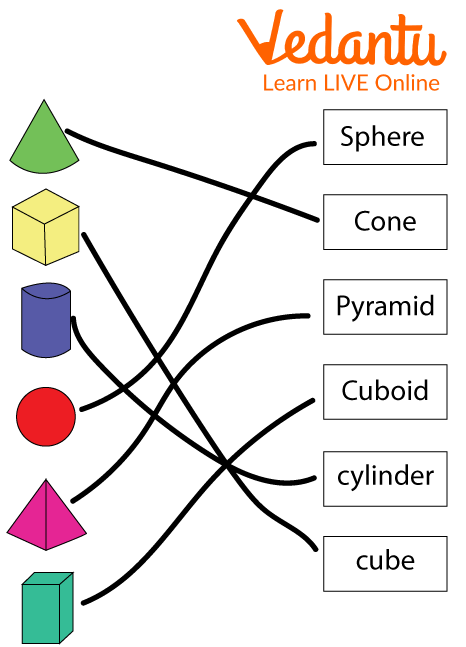## Conclusion:

It may be said that solid geometry permeates every aspect of daily existence. Everywhere is a closed, three-dimensional space. Solid geometry enables us to investigate novel ideas about the forms or figures that we may observe. A solid form is essentially a 3D object, such as a cube, cuboid, cone, etc. We can determine its volume, surface area, and more. In the upcoming section, we will solve and understand the question related to solid geometry.

Last updated date: 21st Sep 2023
Total views: 79.2k
Views today: 2.79k

## FAQs on 3D - Geometry(Solid Geometry)

1. What is the difference between solid and plane geometry?

The most common difference between solid and plane geometry is that the plane geometry is The plane shapes, usually referred to as "2D shapes," are closed, two-dimensional forms with no thickness. Solids with three dimensions: length, width, and height are the only items that constitute a solid form. 3D forms are another name for solid shapes.

2. Who invented solid geometry?

Solid geometry was invented by John Lodge Cowley.

3. What are the main uses of solid geometry?

The uses of solid geometry are as follows,

• Constructions in buildings

• Graphic designing in computers

• Measuring orbit and planetary motions

• Interior designs

4. Why is solid geometry complex to build compared to plane geometry?

Plane geometry has only two dimensions, whereas solid geometry has three dimensions. This is the reason that solid geometry is more complex to build.